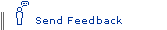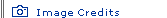Imagining Other Dimensions by Rick Groleau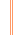The Elegant Universe homepage

For most of us, or perhaps all of us, it's impossible to imagine a world consisting of more than three spatial dimensions. Are we correct when we intuit that such a world couldn't exist? Or is it that our brains are simply incapable of imagining additional dimensions—dimensions that may turn out to be as real as other things we can't detect?

String theorists are betting that extra dimensions do indeed exist; in fact, the equations that describe superstring theory require a universe with no fewer than 10 dimensions. But even physicists who spend all day thinking about extra spatial dimensions have a hard time describing what they might look like or how we apparently feeble-minded humans might approach an understanding of them. That's always been the case, and perhaps always will be.From 2-D to 3-DAn early attempt to explain the concept of extra dimensions came in 1884 with the publication of Edwin A. Abbott's Flatland: A Romance of Many Dimensions. This novel is a "first-person" account of a two-dimensional square who comes to appreciate a three-dimensional world.

The square describes his world as a plane populated by lines, circles, squares, triangles, and pentagons. Being two-dimensional, the inhabitants of Flatland appear as lines to one another. They discern one another's shape both by touching and by seeing how the lines appear to change in length as the inhabitants move around one another.

One day, a sphere appears before the square. To the square, which can see only a slice of the sphere, the shape before him is that of a two-dimensional circle. The sphere has visited the square intent on making the square understand the three-dimensional world that he, the sphere, belongs to. He explains the notions of "above" and "below," which the square confuses with "forward" and "back." When the sphere passes through the plane of Flatland to show how he can move in three dimensions, the square sees only that the line he'd been observing gets shorter and shorter and then disappears. No matter what the sphere says or does, the square cannot comprehend a space other than the two-dimensional world that he knows.

Only after the sphere pulls the square out of his two-dimensional world and into the world of Spaceland does he finally understand the concept of three dimensions. From this new perspective, the square has a bird's-eye view of Flatland and is able to see the shapes of his fellow inhabitants (including, for the first time, their insides).

Armed with his new understanding, the square conceives the possibility of a fourth dimension. He even goes so far as to suggest that there may be no limit to the number of spatial dimensions. In trying to convince the sphere of this possibility, the square uses the same logic that the sphere used to argue the existence of three dimensions. The sphere, now the shortsighted one of the two, cannot comprehend this and does not accept the square's arguments—just as most of us "spheres" today do not accept the idea of extra dimensions.From 3-D to 4-DIt's difficult for us to accept the idea because when we try to imagine even a single additional spatial dimension—much less six or seven—we hit a brick wall. There's no going beyond it, not with our brains apparently.

Imagine, for instance, that you're at the center of a hollow sphere. The distance between you and every point on the sphere's surface is equal. Now, try moving in a direction that allows you to move away from all points on the sphere's surface while maintaining that equidistance. You can't do it. There's nowhere to go—nowhere that we know anyway.

The square in Flatland would have the same trouble if he were in the middle of a circle. He can't be at the center of a circle and move in a direction that allows him to remain equidistant to every point of the circle's circumference—unless he moves into the third dimension. Alas, we don't have the four-dimensionsal equivalent of Abbott's three-dimensional sphere to show us the way to 4-D. (In mathematics, moving into ever higher dimensions is a walk in the park. See Multidimensional Math.)How about 10-D?In 1919, Polish mathematician Theodor Kaluza proposed that the existence of a fourth spatial dimension might allow the linking of general relativity and electromagnetic theory. The idea, later refined by the Swedish mathematician Oskar Klein, was that space consisted of both extended and curled-up dimensions. The extended dimensions are the three spatial dimensions that we're familiar with, and the curled-up dimension is found deep within the extended dimensions and can be thought of as a circle. Experiments later showed that Kaluza and Klein's curled-up dimension did not unite general relativity and electromagnetic theory as originally hoped, but decades later, string theorists found the idea useful, even necessary.

The mathematics used in superstring theory requires at least 10 dimensions. That is, for the equations that describe superstring theory to begin to work out—for the equations to connect general relativity to quantum mechanics, to explain the nature of particles, to unify forces, and so on—they need to make use of additional dimensions. These dimensions, string theorists believe, are wrapped up in the curled-up space first described by Kaluza and Klein.

To extend the curled-up space to include these added dimensions, imagine that spheres replace the Kaluza-Klein circles. Instead of one added dimension we have two if we consider only the spheres' surfaces and three if we take into account the space within the sphere. That's a total of six dimensions so far. So where are the others that superstring theory requires?

It turns out that, before superstring theory existed, two mathematicians, Eugenio Calabi of the University of Pennsylvania and Shing-Tung Yau of Harvard University, described six-dimensional geometrical shapes that superstring theorists say fit the bill for the kind of structures their equations call for. If we replace the spheres in curled-up space with these Calabi-Yau shapes, we end up with 10 dimensions: three spatial, plus the six of the Calabi-Yau shapes, plus one of time.

If superstring theory turns out to be correct, the idea of a world consisting of 10 or more dimensions is one that we'll need to become comfortable with. But will there ever be an explanation or a visual representation of higher dimensions that will truly satisfy the human mind? The answer to this question may forever be no. Not unless some four-dimensional life-form pulls us from our three-dimensional Spaceland and gives us a view of the world from its perspective.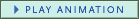Creatures living in Flatland see triangles and other two-dimensional objects as lines.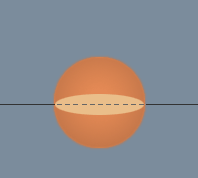From a two-dimensional point of view, a sphere passing through a plane appears as a line that initially gets longer, then, as this image shows, gets shorter and shorter until it disappears altogether.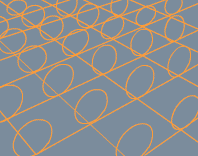The circles represent an additional spatial dimension that is curled up within every point of our familiar three-dimensional space.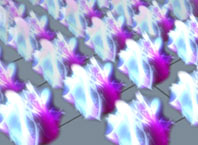Six-dimensional Calabi-Yau shapes can account for the additional dimensions required by superstring theory.Back to the Elegant Universe homepage for more articles, interviews, interactives, and slide shows.

 Rick Groleau is managing editor of NOVA online.